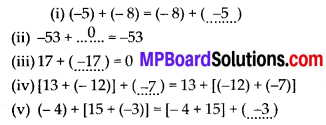# MP Board Class 7th Maths Solutions Chapter 1 Integers Ex 1.2

## MP Board Class 7th Maths Solutions Chapter 1 Integers Ex 1.2

Question 1.
Write down a pair of integers whose:
(a) sum is -7
(b) difference is -10
(c) sum is 0
Solution:
(a) – 9 + (2) = – 7
(b) – 14 – (-4) = – 10
(c) 6 + (-6) = 0

Question 2.
(a) Write a pair of negative integers whose difference gives 8.
(b) Write a negative integer and a positive integer whose sum is -5.
(c) Write a negative integer and a positive integer whose difference is -3.
Solution:
(a) -3 – (-11) = 8
(b) -10 + 5 = -5
(c) -1 – (2) = – 3Question 3.
In a quiz, team A scored – 40,10,0 and team B scored 10, 0, -40 in three successive rounds. Which team scored more? Can we say that we can add integers in any order?
Solution:
Team A scored – 40, 10, 0.
Total score of team A = – 40 + 10 + 0 = – 30
Team B Scored 10, 0, -40.
Yes, we can add integers in any order. We had observed that the scores obtained by both teams in successive rounds were numerically equal but different in order. Yet, the total score of both teams were equal.Question 4.
Fill in the blanks to make the following statements true:
(i) (-5) + (- 8) = (- 8) + (….)
(ii) -53 + …… = -53
(iii) 17 + ….. = 0
(iv) [13 + (- 12)] + (…… ) =13 + [(-12) + (-7)]
(v) (- 4) + [15 + (-3)] = [-4 + 15] + …..
Solution: